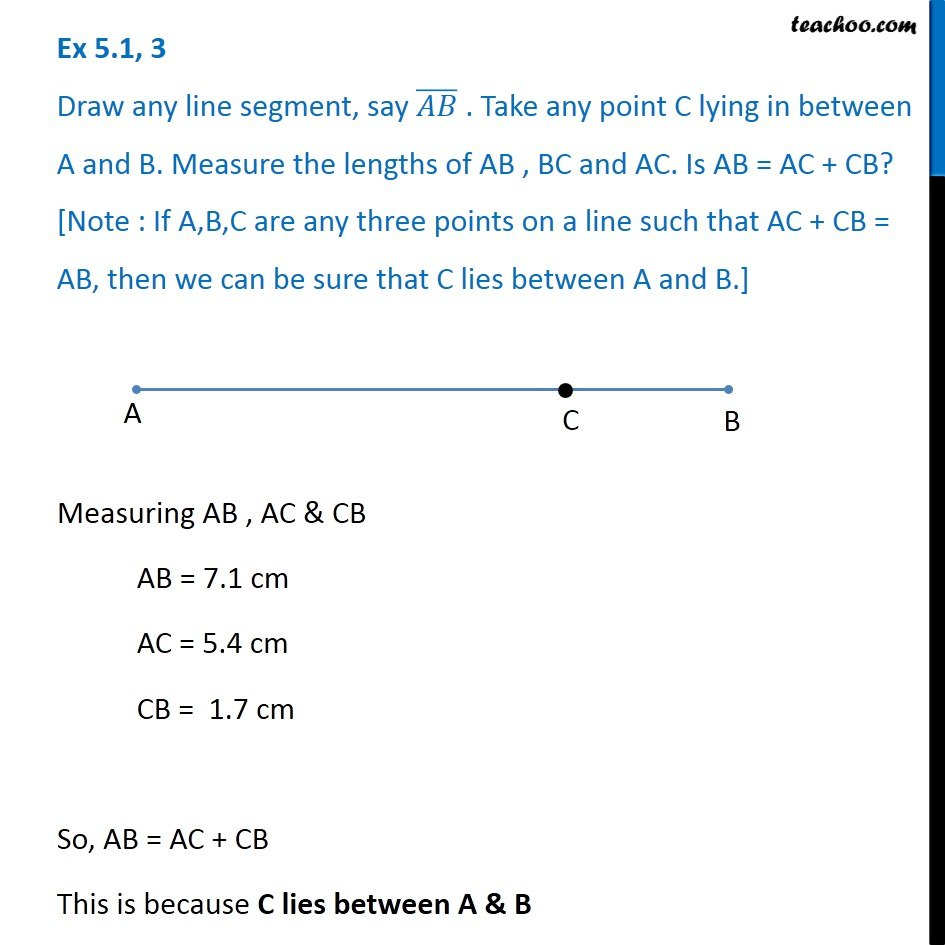1. Chapter 5 Class 6 Understanding Elementary Shapes
2. Concept wise
3. Measuring Line Segments

Transcript

Ex 5.1, 3 Draw any line segment, say (𝐴𝐵) ̅ . Take any point C lying in between A and B. Measure the lengths of AB , BC and AC. Is AB = AC + CB? [Note : If A,B,C are any three points on a line such that AC + CB = AB, then we can be sure that C lies between A and B.] Measuring AB , AC & CB AB = 7.1 cm AC = 5.4 cm CB = 1.7 cm So, AB = AC + CB This is because C lies between A & B

Measuring Line Segments